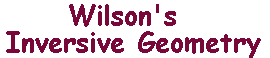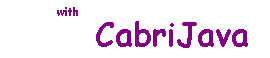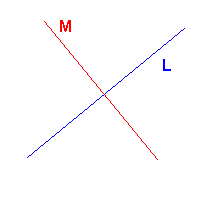The Algebraic Inversion Theorem

 We use complex numbers as "coordinates" in E. The point A(a,b) is described by the complex number a =a + ib. Then the locus Ak(A,B) = {P : PA = k.PB} has equation |z -a| = k.|z - b|, where a, b are the coordinates A, B. For typographical reasons, we will use z* to denote the complex conjugate of z. Immediately we see that inversion in the i-line given by the real axis is reflection in the real axis, i.e. maps z to z* (and ∞ to itself). We shall simply say that A is the point a Inversion in the i-line |z| = r is also easy to describe, it maps z to r2/z* (non-zero z), and interchanges 0 and ∞. We are now in a position to give a neat proof that inversion maps i-lines to i-lines. As a bonus, we get information about the images of inverse points. The Algebraic Inversion Theorem Suppose that L and C are i-lines, and that iC denotes inversion with respect to C. Then iC(L) is an i-line, if A and B are inverse with respect to L, then iC(A) and iC(B) are inverse with respect to iC(L). iC° iL = iL'° iC ,where L' = iC(L). We can now prove a result fundamental to the creation of hyperbolic geometry. Observe that, if L and M are lines, then iL is just reflection in L. It follows that iL(M) = M if and only if L and M are orthogonal. In fact, this is true for any i-lines. As you may guess, the proof amounts to showing that the general case can be reduced to this special case by applying inversion. The Mirror Property Suppose that L and M are distinct i-lines, then iL(M) = M if and only if L and M are orthogonal.In fact, when , L and M are orthogonal, more is true. If L and M are orthogonal i-lines, then iL(Mº) = Mº, where Mº is the interior of M. In the CabriJava pane on the right, S is a point on the arc of L lying inside M. P is the centre of L, and PS cuts M at R and T. Then i(R) = T since iL maps M to M, and the i-line PS to itself. Also iL(S) = S (on L). A lies on the segment RS, so its inverse A' lies on ST. Thus the points of Mº inside L map to those outside and vice versa. You can experiment by dragging S or A. We can think of this as saying that the two regions of the disc Mº are "reflections" of one another in a circular "mirror" L. This is not optically sound, but it is a good analogy.